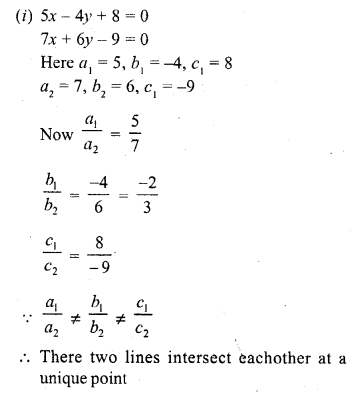• +91 9971497814
• info@interviewmaterial.com

# RD Chapter 3- Pair of Linear Equations in Two Variables Ex-3.1 Interview Questions Answers

### Related Subjects

Question 1 : Akhila went to a fair in her village. She wanted to enjoy rides on the Giant Wheel and play Hoopla (a game in which you throw a rig on the items kept in the stall, and if the ring covers any object completely you get it). The number of times she played Hoopla is half the number of rides she had on the Giant Wheel. Each ride costs Rs. 3, and a game of Hoopla costs Rs. 4. If she spend Rs. 20 in the fair, represent this situation algebraically and graphically.

Let number of rides on the wheel = x
and number of play of Hoopla = y
According to the given conditions x = 2y ⇒ x – 2y = 0 ….(i)
and cost of ride on wheel at the rate of Rs. 3 = 3x
and cost on Hoopla = 4y
and total cost = Rs. 20
3x + 4y = 20 ….(ii)
Now we shall solve these linear equations graphically as under
We take three points of each line and join them to get a line in each case the point of intersection will be the solution
From equation (i)
x = 2y
 X 4 0 6 y 2 0 3
y = 2, then x = 2 x 2 = 4
y = 0, then x = 2 x 0 = 0
y = 3, then x = 2 x 3 = 6
Now, we plot these points on the graphs and join them to get a line
Similarly in equation (ii)
3x + 4y = 20 ⇒ 3x = 20 – 4y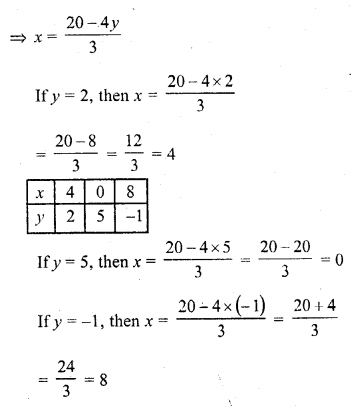Now we plot these points and get another line by joining them
These two lines intersect eachother at the point (4, 2)
Its solution is (4, 2)
Which is a unique Hence x = 4, y = 2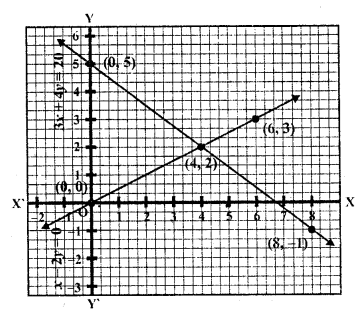Question 2 : Aftab tells his daughter, “Seven years ago, I w as seven times as old as you were then. Also, three years from now, I shall be three times as old as you will be.” Is not this interesting ? Represent this situation algebraically and graphically.

Seven years ago
Let age of Aftab’s daughter = x years
and age of Aftab = y years
and 3 years later
Age of daughter = x + 10 years
and age of Aftab = y + 10 years
According to the conditions,
y = 7x ⇒ 7x – y = 0 ……….(i)
y + 10 = 3 (x + 10)
=> y + 10 = 3x + 30
3x – y = 10 – 30 = -20
3x – y = -20 ….(ii)
Equations are
7x – y = 0
3 x – y = -20
Now we shall solve these linear equations graphically as under
7x – y = 0 ⇒ y = 7x
 X 0 1 -1 y 0 7 -7
If x = 0, y = 7 x 0 = 0
If x = 1, y = 7 x 1=7
If x = -1, y = 7 x (-1) = -7
Now plot these points on the graph and join
then
3x – y = -20
y = 3x + 20
 X -1 -2 -3 y 17 14 11
If x = -1, y = 3 x (-1) + 20 = -3 + 20= 17
If x = -2, y = 3 (-2) + 20 = -6 + 20 = 14
If x = -3, y = 3 (-3) + 20 = -9 + 20= 11
Now plot the points on the graph and join them we see that lines well meet at a point on producing at (5, 35).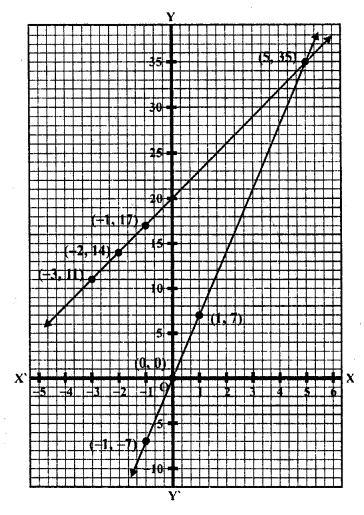Question 3 : The path of a train A is given by the equation 3x + 4y – 12 = 0 and the path of another train B is given by the equation 6x + 8y – 48 = 0. Represent this situation graphically.

Path of A train is 3x + 4y – 12 = 0
and path of B train is 6x + 8y – 48 = 0
Graphically, we shall represent these on the graph as given under 3x + 4y- 12 = 0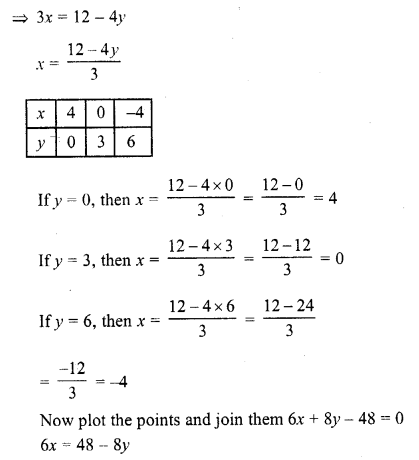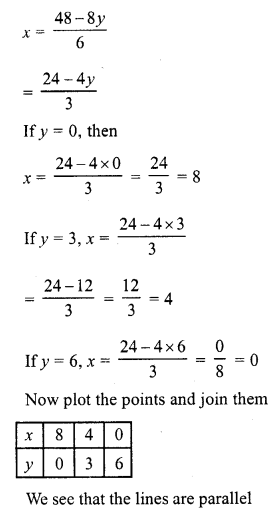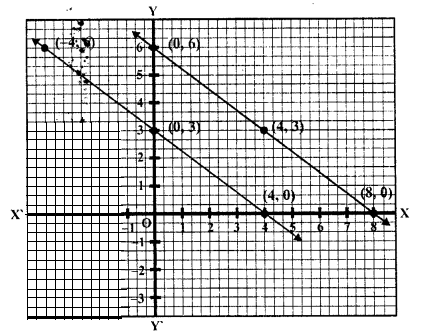Question 4 : Gloria is walking along the path joining (-2, 3) and (2, -2), while Suresh is walking along the path joining (0, 5) and (4, 0). Represent this situation graphically.

Plot the points (-2, 3) and (2, -2) and join them to get a line
and also plot the points (0, 5), (4, 0) and joint them to get another line as shown on the graph
We see that these two lines are parallel to each other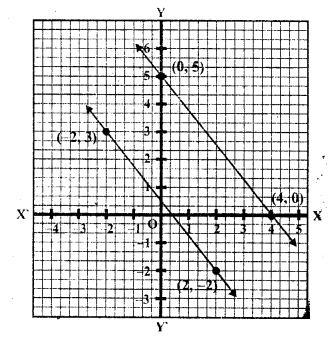Question 5 :
On comparing the ratios , and without drawing them, find out whether the lines representing following pairs of linear equations intersect at a point, are parallel or coincide :
(i) 5x – 4y + 8 = 0
7x + 6y – 9 = 0
(ii) 9x + 3y +12 = 0
18x + 6y + 24 = 0
(iii) 6x – 3y +10 = 0
2x – y + 9 = 0